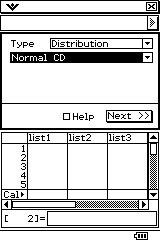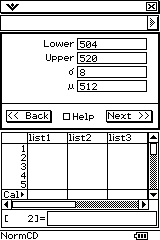Showing posts with label Classpad 330. Show all posts
Showing posts with label Classpad 330. Show all posts

## Tuesday, April 3, 2012

### CAS calculator and differentiation

There are a host of ways to find the first derivative on the CAS calculator and to solve calculus problems quickly.  Sometimes I think exam writers are well behind what these calculators can do and fail to understand how trivial some problems have become.

a) Find y at x=3 for y = 2x^2 + 2x + 2

The "|" is important between the equation y = 2x^2 + 2x + 2 and x=3

Let's now find x if we know y.  This is a little harder as we have to solve the equation.

b) Find x at y=14 for y = 2x^2 + 2x + 2The easiest way to do this is to type 14 = 2x^2 + 2x + 2, highlight it using your stylus (this is important!) and then go

Note that it find both possible solutions (unlike using numsolve with the incorrect range specified)

Let's find the first derivative.  For this use the 2D template in the soft keyboardGo keyboard -> 2D -> Calc ->

c) Find the 1st derivative of y=2x^2 + 2x + 2

Note that I removed the "y=" this time.  I differentiated the expression on purpose as it makes the next part easier.

Finding the 1st derivative/gradient/instantaneous rate of change at a point is also easy.

d) Find the 1st derivative of y = 2x^2 + 2x + 2 at x = 3

As you can see, it is a mix between c) and a)

Last but not least we can find a point for a particular gradient.

e) Find x at y' = 14 for y = 2x^2 + 2x + 2To find y itself we could repeat a)

It's very much a case of thinking what you need and then finding it.  As you can see it can all be done with one line on a CAS calculator, things that would take multiple steps on paper.  TanLine is also a useful function that can be investigated and used to quickly find tangents.

Viola!

## Sunday, August 23, 2009

### Moving averages and the CAS calculator

On the fx casio, moving averages were a bit of a doddle.. stick in the values and use the MAV application.

The suggested method for the classpad 330 according to the classpad website is to use spreadsheet mode. This has some disadvantages that I have not been able to overcome with my brief investigation.

To set up a MAV problem, clear the sheet and enter the basic data in columns. To calculate the MAV click on the middle cell of the first series (eg in a 3pt MAV it would be the second cell) and use the Action column to find the mean of the first three data cells. Then copy your mean cell down the column by highlighting the rest of the column using the stylus and then use the edit menu -> paste.

To graph it is a little on the fiddly side. Highlight the data column and the MAV column and press the line graph icon (there are two, it's the first one). Select the graph and go View->Markers to turn off the data markers. Under the Type menu, make sure you have selected Column Series.

This should display the graph.

If you need a line of regression, select the MAV line with the stylus and select an appropriate regression function on the toolbar. The main issue with it is that there is no way to interpolate or extrapolate data (the main thing you wish to do with this sort of data) for predicting data points whilst in spreadsheet mode for the line of regression. It's been fairly frustrating. I can recreate the line of regression for the MAV in "graph and tab" mode and use this but it would be easier just to have a trace mode on the stats graph... oh well...

Here's a link to an index of other CAS calculator posts.

Update 15/09/09: Download v3.04 of the classpad operating system from here. It greatly enhances the ability of spreadsheet mode to perform regression in spreadsheet mode. You will need to register and login before downloading it.  It's quite a neat trick to click on the line of regression, copy it and then paste the formula onto the spreadsheet at the the bottom of the y column.  Modify the formula such that x becomes a cell reference (eg A2) and viola - an alternate to trace for predicting y values.

:-)

## Tuesday, January 20, 2009

### Solving Simultaneous Equations using the Classpad 330

There are a number of ways of finding where two lines intersect. Let's solve this example.

"Where do the equations y=x and y=-2x+3 intersect?"
One way to find the solution is to solve the two equations algebraically using simultaneous equations.

First open and clear the main work pane.Press the blue Keyboard button and bring up the soft keyboard. Select the 2D tab.

You should be able to see a button with a bracket and two small boxes (circled below in red). Press it.

You should see the simultaneous equation template in the main pane.
(Update 1/6/2010: Press it twice to add a third equation line!)
Click on the box below it and type y=-2x+3

In the third box to the right of the vertical line type x,y (the variables we wish to solve).

Hit the exe button.

The answer (x=1, y=1) should appear.

Here is a link to other CAS calculator posts.

## Thursday, January 8, 2009

### Classpad 330 and Normal Distribution

Normal distribution problems can be done quite simply on the Classpad 330, but the method seems a little weird.. perhaps I haven't found a menu yet, or completed an update.. but here's how I did it for the following problem.
"A packet of mince contains 500g of mince. Suppose the actual weight (x) of
these packets is normally distributed with a mean of 512 grams and a standard
deviation of 8 grams. What is the probability of picking a packet between 504
and 520g?"
Firstly open the main window and add the list editor from the toolbar.

Opening the list editor should make the Calc menu appear in the menubar at the top of the window.

Select Calc->Distribution. This will make a popup appear with some options

The Type dropdown needs to say Distribution.

The second dropdown should say Normal CD. After that is selected tap Next. A new dialog box will appear.For our problem the lower bound is 504, the upper bound is 520, the standard deviation is 8 and the mean is 512. Tap Next when this has been entered. The answer will appear with a probability of 0.683Tap the graph icon in the toolbar to view the distribution.Viola!
Here is a link to other CAS calculator posts.

## Sunday, October 19, 2008

### My Casio Classpad 330 JourneySecond weekend playing with the calculator.

When I was doing phone support often I could not see what the person on the other end was doing. I became quite adept at directing customers on quite difficult tasks blind. The most important thing to do was to adequately define things up front.

With the CAS calculator the windowing system can be quite confusing at first. It is important to name things in such a way that students can listen to your direction and follow it, rather than needing snapshots all of the time.

In the worksheets I have created, the calculator is divided into the screen and the buttons. The screen in divided into the menu bar, the tool bar, the work pane, the status bar and the icon panel. The buttons are blue, grey and black.

i.e.
The Edit, Action, Interactive text at the top is the menu bar
The icons underneath the menu bar is the tool bar
The area underneath is the work pane, it can be split into the top pane and the bottom pane. The work pane is currently filled with the main application.
The bit beneath the work pane (eg. Alg, Standard, Real, Deg, battery indicator) is the status bar.
The stylus and buttons are used to enter data and operations into the calculator.

Here's a link to the "How do I.. ???? on a Casio Classpad" book that I have been using.
Here's a link back to my first article on CAS calculators
Here's a link to an index of other CAS calculator posts.